In one of the previous article, we provided Javascript Unit testing frameworks, which are front-end Unit testing framework, but what about back-end C# unit testing, so in this article, I am going to explain what is unit testing and how we can apply unit testing in C# using Visual Studio with a simple example.

### What is Unit Testing?

Software applications are built using various small functions, which are dependent on each other to create a bigger application.

If we check each function output of individual components, then it will become a very complex task to check the output of each function for large projects.

There we should have automated tests to run, whenever application functions changes, to check if the working still remains intact or not.

So to make it easier, we write some test cases for individual components of the application, to check if the code is working as needed and giving us expected output with sample values or not, if the test is giving correct output it is marked as "passed" else "failed."

This testing is called "Unit testing" because we break down the application functionality into smaller functions and test them individually, which can be called units.

### Importance of using Unit testing

• Unit testing gives more reliable code
• Reusable codes reduce the effort and save time as the code needs to be modular in unit testing.
• Unit Testing helps in easy debugging, if any new test fails, you can easily debug the error in your code.
• Provides Documentation: Unit testing helps in creating software documentation, so if any software developer joins your team, he/she can easily understand the system using a Unit test.
• Unit testing allows software developers to actually think through the design of the software and what has to be done before they write the code.
• Reduces Cost, while using Unit testing, we can get the error in the code in the early stage of development, which saves time and reduce code of development.

### Unit testing example in C# using MS test in Visual Studio

Now, we know the basics and advantages of unit testing, we can proceed to the unit testing example in C# using MS tests in Visual Studio.

In this test, we will be using a console application project and will add a new unit test project in the same solution.

Basically, we will create Unit Tests for the sample class method of adding two numbers.

Step 1: Open your Visual Studio and create a console application by navigating to "File" -> "New" -> "Project" -> Select "Window Desktop" from left-pane and "Console application" from right-pane, name it "AddNumber" and then click "Ok"

Step 2: In your project, add a new class by right-clicking on Project and then select "Add"-> "New" -> "Class", name it as "Numbers.cs" and use the below code

``````namespace AddNumber
{
public class Numbers
{

public int Add(int num1, int num2)
{
int num3 = num1 + num2;
return num3;
}
}
}
``````

Step 3: Now, we have the basic Console application, let's add a new Unit Test project in the same solution.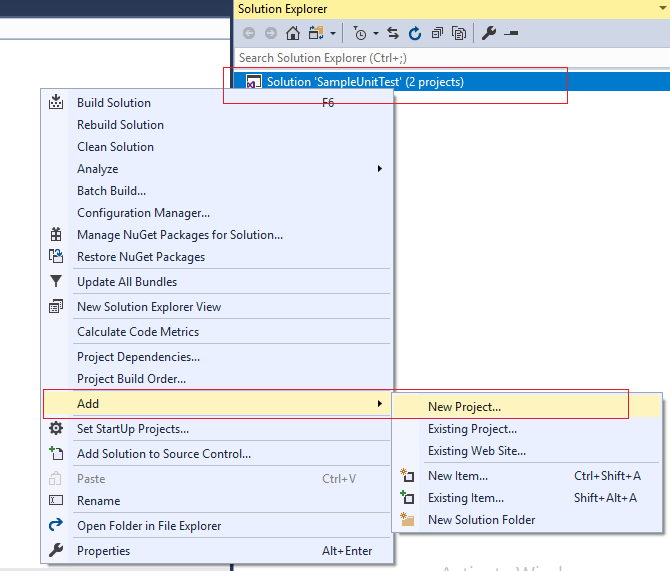Now, select "Test" From the left-pane and "Unit test" from the right-pane, name your project, and click Ok, as shown in the below image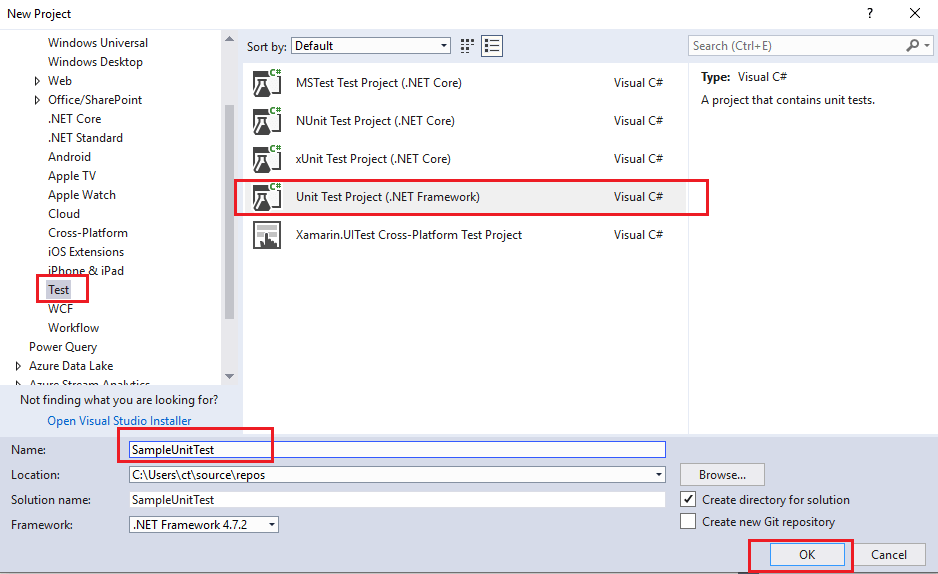Step 4: You will see we have already created a Unit test class with below sample code

``````namespace SampleUnitTest
{
[TestClass]
public class UnitTest2
{
[TestMethod]
public void TestMethod1()
{
}
}
}
``````

But before we write our own code, you need to add a reference to the main console application (you need to build the console application before adding its reference).

So add it by right-clicking on "Reference" inside Unit test project-> Select "Add-reference"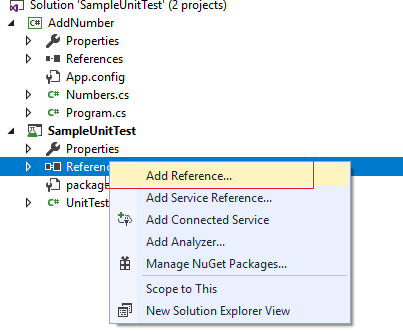Then select "Projects"-> "Solution", and click "Ok" to add a reference.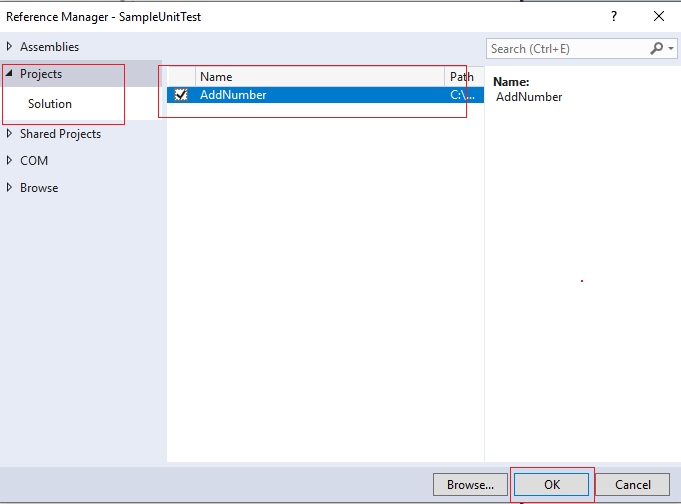Step 5: Now use the below code in "`UnitTest1.cs`" to write Unit tests for "Add" method

``````using AddNumber;
using Microsoft.VisualStudio.TestTools.UnitTesting;

namespace SampleUnitTest
{
//Unit test class
[TestClass]
public class CheckResultIsPositive
{
[TestMethod]
public void CheckResult()
{
var num = new Numbers();

int result = num.Add(10, 15); //will add both numbers

Assert.IsTrue(result > 0); //check if result is positive or not
}

//this test will check if, when adding numbers using Numbers.cs -> Add method, output is as expected or not
[TestMethod]
public void CheckIfOutputIsExpected()
{
var num = new Numbers();

int result = num.Add(20, 10); //will add both numbers

Assert.AreEqual<int>(30, result); //check if result is equal to expected value 30
}
}
}
``````

In the above code, we have created 2 Test Methods

Test Method 1: Create an object of type "Numbers" class and then use its method to add two numbers using "`num.Add()`", which then checks if the output is greater than 0 or not.

If not, the test will not be passed, but as values are "`num.Add(10,15)`" and result="25" which is greater than 0, so it passes the test.

`Assert.True(Condition)` Method in above code, check if the condition is true, if yes, then test is considered as passed.

Test Method 2: This Test method again creates an object of type "Numbers" class and then use its method to add two numbers using "`num.Add()`", which then checks if the result is coming as expected.

As we are adding two numbers "num.Add(20, 10);", so expected result is "30".

So, using `Assert.AreEqual<int>(30, result);` code we check if the method is returning expected output or not.

You can run these tests and check if the Tests are passed or not.

First, open "Test Explorer" by navigating to Test -> Windows -> Test Explorer (or press Ctrl + E, T)

Then click on "Run All", you will see the output as below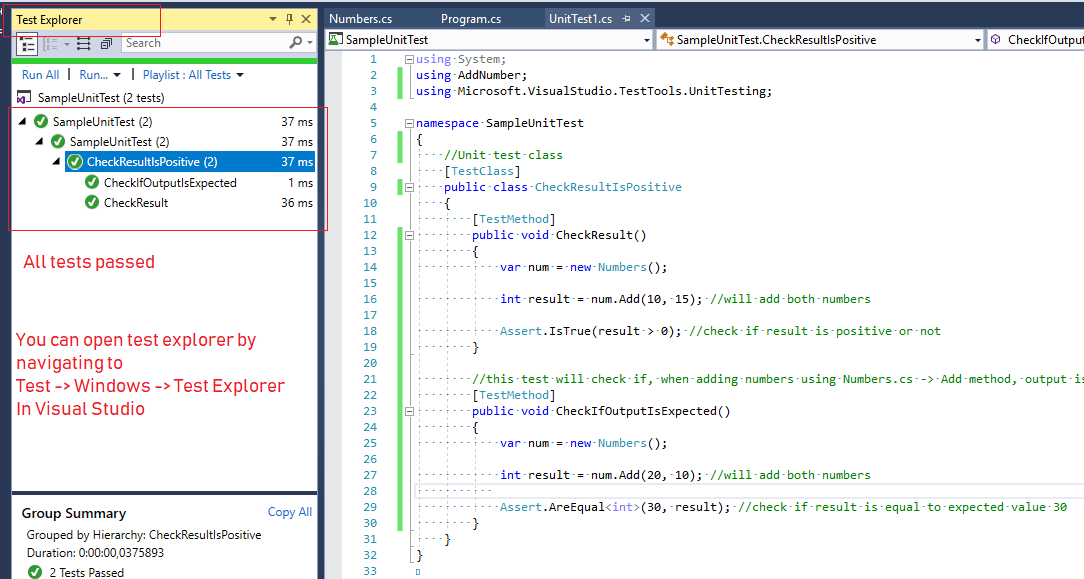Once all test runs, bar turns green if all the test methods pass, or red if any of the tests fail.

If any test fails, most probably, your main C# code related to "Add" Method in Number.cs.

So that's it, these were basics of Unit Testing in C#.

You will need to use Assert Class methods appropriately to create your Unit Test Methods.

You may also like to read:

C# Regex Example and Basics

Best Open Source APM Tools

What is responsive web design and it's importance